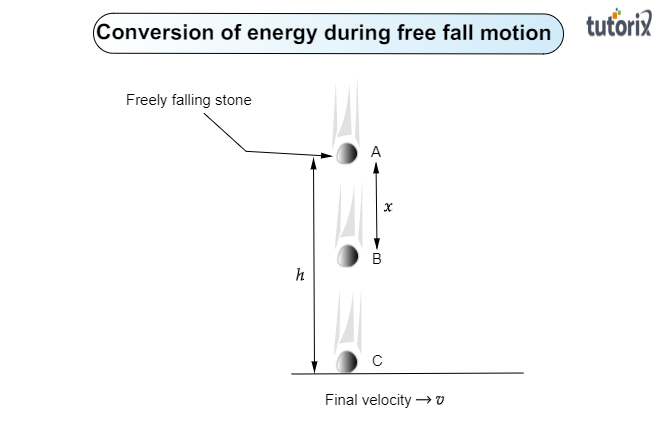# The potential energy of a freely falling object decreases progressively. Does this violate the law of conservation of energy? Why?

Given:

Often, it is found that the potential energy of a freely falling object decreases progressively.

To do:

We have to determine whether it violates the law of conservation of energy.

Solution:

To determine whether a progressively decreasing potential energy of a freely falling object does not violate the Law of Conservation of Energy let us know a little about the law of the conservation of energy:

Law of energy conservation:

"The Law of energy conservation states that energy can neither be created nor can be destroyed. Energy can be transformed only from one form to another form."

Energy conservation of a freely falling object:

Let us check the energy conservation of a freely falling object of mass $m$ that is dropped from a certain height $h$. When it is dropped from point A and reaches the ground at point C. Let us calculate its total energy at various points A, B, and C:At point A:

When the object is dropped from a height $h$ its initial velocity is zero.

$v_A=0$

Therefore, its kinetic energy, $K_A=\frac{1}{2}mv^2=0$

Potential energy, $P_A=mgh$

Total energy, $E_A=K_A+P_A=0+mgh=mgh$

At point B:

At point B, let $v_B$ its velocity and it covered distance $x$ vertically.

On using the second equation of motion, $v_B^2=u^2+2gx$

Or $v_B^2=0^2+2gx=2gx$

Therefore, its kinetic energy, $K_B=\frac{1}{2}mv_B^2$

$=\frac{1}{2}\times m\times 2gx=mgx$

Now its height is $(h-x)$ at point B.

Therefore, its potential energy, $P_B=mg(h-x)$

Total energy of the object, $E_B=P_B+K_B$

$=mg(h-x)+mgx$

$=mgh-mgx+mgx$

$=mgh$

Therefore, the total energy of the freely falling object remains the same at point B.

At point C:

When the freely falling object reaches at the ground, its height becomes zero.

Therefore, it potential energy, $P_C=mg\times 0=0$

Its velocity becomes $v$, on using the second equation of the motion:

$v^2=u^2+2gh$

Or $v^2=0+2gh=2gh$

Its kinetic energy, $K_C=\frac{1}{2}\times m\times 2gh$

$=mgh$

At, the total energy of the freely falling object remains the same at point C also.

On comparing the total energy of the freely falling object at various points A, B, and C:

We find that $E_A=E_B=E_C$

The total energy of the freely falling object remains the same at each point A, B, and C. This proves the law of energy conservation.

Conclusion:

Therefore, When a body falls freely Potential energy starts decreasing due to loss of Height, at the same time Kinetic energy starts increasing due to an increase in velocity. And the sum of Potential energy and kinetic energy remains constant. The potential energy of a freely falling object decreases progressively. It does not violate the law of conservation of energy.

Updated on: 10-Oct-2022

112 Views